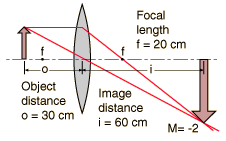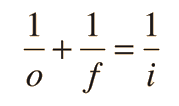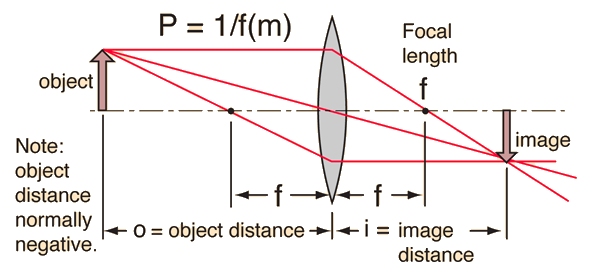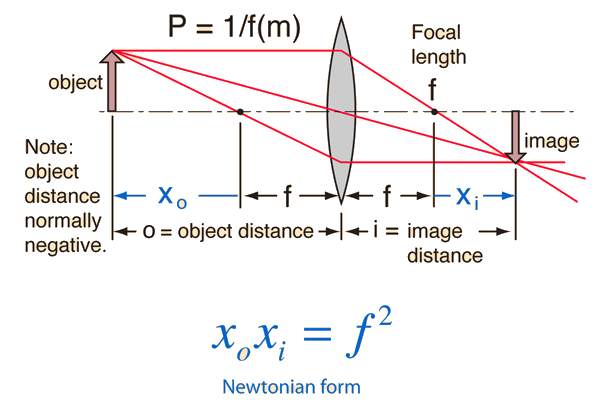# Thin Lens Equation

A common Gaussian form of the lens equation is shown below. This is the form used in most introductory textbooks. A form using the Cartesian sign convention is often used in more advanced texts because of advantages with multiple-lens systems and more complex optical instruments. Either form can be used with positive or negative lenses and predicts the formation of both real and virtual images. It is valid only for paraxial rays (rays close to the optic axis) and does not apply to thick lenses.Enter data below, then click on the quantity you wish to calculate in the active formula above.
 For a lens of focal length f = cm, corresponding to lens power P = diopters, an object distance of o = cm will produce an image at i = cm. The linear magnification will be M = .If the lens equation yields a negative image distance, then the image is a virtual image on the same side of the lens as the object. If it yields a negative focal length, then the lens is a diverging lens rather than the converging lens in the illustration. The lens equation can be used to calculate the image distance for either real or virtual images and for either positive on negative lenses. The linear magnification relationship allows you to predict the size of the image.

 Form of lens equation with Cartesian sign convention Reversibility of object and image points: conjugate points
Index

Lens concepts

 HyperPhysics***** Light and Vision R Nave
Go Back

# Thin-Lens Equation:Cartesian Convention

If the Cartesian sign convention is used, the Gaussian form of the lens equation becomesbecause in that convention the positive direction for a quantity is always in the direction that light is traveling. The object distance o is then a negative number because to travel from the lens to the object, you must travel in the direction opposite to light travel. The thin lens equation is also sometimes expressed in the Newtonian form. The derivation of the Gaussian form proceeds from triangle geometry. For a thin lens, the lens power P is the sum of the surface powers. For thicker lenses, Gullstrand's equation can be used to get the equivalent power.To common form of lens equation in introductory texts
Index

Lens concepts

 HyperPhysics***** Light and Vision R Nave
Go Back

# Cartesian Sign Convention

1. All figures are drawn with light traveling from left to right.
2. All distances are measured from a reference surface, such as a wavefront or a refracting surface. Distances to the left of the surface are negative.
3. The refractive power of a surface that makes light rays more convergent is positive. The focal length of such a surface is positive.
4. The distance of a real object is negative.
5. The distance of a real image is positive.
6. Heights above the optic axis are positive.
7. Angles measured clockwise from the optic axis are negative.

Because the direction of light travel is consistent and there is a consistent convention to determine the sign of all distances in a calculation, this sign convention is used in many texts. It has some advantages when dealing with multilens systems and more complex optical instruments.

 Cartesian sign convention applied to: Lens equation Surface power
Index

Lens concepts

Meyer-Arendt, 2nd Ed
Sec 1.3

 HyperPhysics***** Light and Vision R Nave
Go Back

# Thin-Lens Equation:Newtonian Form

In the Newtonian form of the lens equation, the distances from the focal length points to the object and image are used rather than the distances from the lens.Newton used the "extrafocal distances" xo and xi in his formulation of the thin lens equation. It is an equivalent treatment, but the Gaussian form will be used in this resource.

 To common form of lens equation in introductory texts
Index

Lens concepts

 HyperPhysics***** Light and Vision R Nave
Go Back